# In-cell 5 star chart – tutorial & template

Share

Whenever we talk about product ratings & customer satisfaction, 5 star ratings come to our mind. Today, let’s learn how to create a simple & elegant 5 star in-cell chart in Excel. Something like this: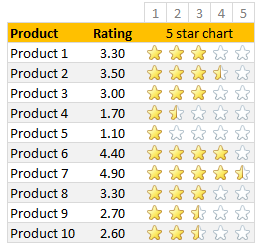A while ago, Hui showed us a fun way to create 5 star charts in Excel using bar charts with 5 star mask. I highly recommend reading that article if you want to create a regular chart version of this.

## Tutorial for creating a 5 star chart

### 1. Meet the data

Here is our data. Very simple. First column has product names. Second column has customer rating – from 1 to 5.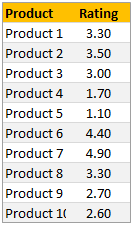### 2. Set up 5 blank columns for the 5 star chart

Let’s create a 5 column grid right next to our data set. This is where the in-cell 5 star chart will go. At this stage our 5 star chart looks like this:If you haven’t guessed yet, we will be using conditional formatting > star icons to get the 5 star chart.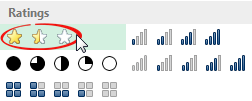### 3. Write formulas in the 5 column grid

Now, we need to write formulas to fill up the 5 column grid. We need to formulas to return either 1, 0 or decimal values in the grid depending on the rating for that row.

So, for example, if a product has 3.30 rating, we want to print 1, 1, 1, 0.30 and 0 in 5 columns.

You can use any number of formulas to get this result. The simplest one will be IF formula.

Assuming column C (from C7) has product ratings & row 5 has running numbers 1 to 5 (from cell D5), we can use below formula to get what we want:

=IF(D\$5<=\$C7,1,IF(ROUNDUP(\$C7,0)=D\$5,MOD(\$C7,1),0))

To understand the above formula , see this illustration.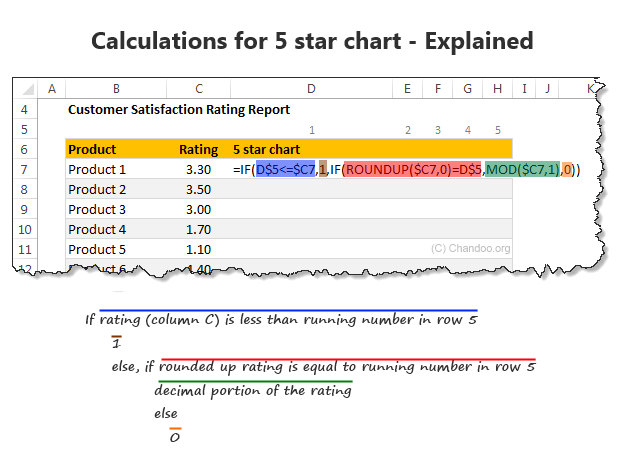If you like to avoid IF formulas, here is an alternative:

=MAX((\$C7>=D\$5)*1,MOD(\$C7,1))*(ROUNDUP(\$C7,0)>=D\$5)

A challenge for you: Can you think of any other ways to write this formula?

### 4. Apply conditional formatting to the 5 column grid

Select the 5 column grid and apply conditional formatting (Home > Conditional Formatting > New rule)

Set up the rule as shown below: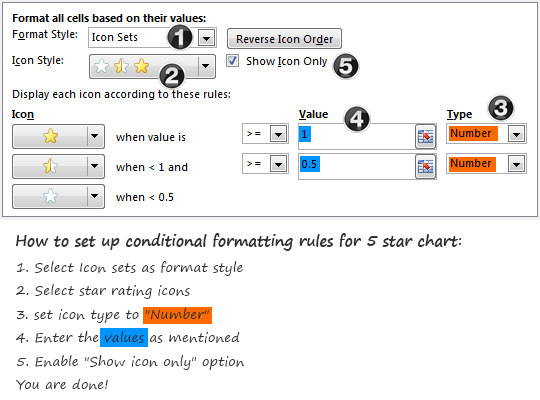At this stage, our report looks like this: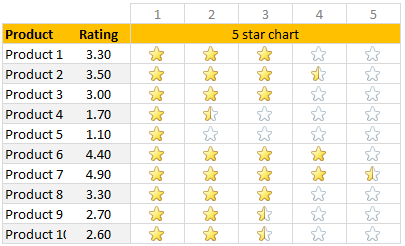### 5. Adjust column width and borders

Once the formatting is applied, just clean up the report by adjusting column width (set it to 24 px) and add horizontal borders only.

And our product rating report is ready.## Download in-cell 5 star chart template

Please click here to download the in-cell 5 star chart workbook. It also contains a variation of the 5 star chart made with data bars & 5 star mask. Check out both examples to understand how they work.

## More in-cell chart tutorials & techniques

In-cell charts are a powerful & lightweight way to visualize your data. Check out below tutorials to one up your awesomeness.

## How would you visualize customer ratings in Excel?

While 5 star charts are traditional, they dumb-down the data. Can you think of other fun ways to visualize customer / product rating data? Please share your thoughts & implementations in the comments.

### Get FREE Excel + Power BI Tips

Simple, fun and useful emails, once per week.

Learn & be awesome.

### Welcome to Chandoo.org

Thank you so much for visiting. My aim is to make you awesome in Excel & Power BI. I do this by sharing videos, tips, examples and downloads on this website. There are more than 1,000 pages with all things Excel, Power BI, Dashboards & VBA here. Go ahead and spend few minutes to be AWESOME.

Read my storyFREE Excel tips book

Chandoo is an awesome teacher
5/5

– Jason

### 100 Excel Formulas List

From simple to complex, there is a formula for every occasion. Check out the list now.

### 20 Excel Templates

Calendars, invoices, trackers and much more. All free, fun and fantastic.### Weighted Average in Excel [Formulas]

Learn how to calculate weighted averages in excel using formulas. In this article we will learn what a weighted average is and how to Excel’s SUMPRODUCT formula to calculate weighted average / weighted mean.

What is weighted average?

Wikipedia defines weighted average as, “The weighted mean is similar to an arithmetic mean …, where instead of each of the data points contributing equally to the final average, some data points contribute more than others.”

Calculating weighted averages in excel is not straight forward as there is no built-in formula. But we can use SUMPRODUCT formula to easily calculate them. Read on to find out how.

## Related Tips

### 11 Responses to “In-cell 5 star chart – tutorial & template”

1.Jan Bolhuis says:

=IF(\$C8>=D\$6,1,IF(MOD(\$C8,1)+\$C8=D\$6,0,5,0))

To hide the empty stars:

=IF(\$C8+0,5=D\$6,1,IF(MOD(\$C8,1)+\$C8=D\$6,0,5,0)))

2.sam says:

In cell D7 type =\$C7-D\$5+1 and copy paste the formula

•Jan Bolhuis says:

Great! And afterwards very logical. Thank you.

•Chandoo says:

Hi Sam.. that is genius. Very elegant solution indeed. Thanks for posting it 🙂

3.Ramesh says:

Great! Thank you

4.Hocine Satour says:

Thank you Chandoo

5.Jervilicious says:

Hi! Does this only work for Excel 2015? thanks!

6.Squalle says:

These are all great solutions. Just one thing that was a little confusing (probably more so for newer excel users).

In the explanation, you have:
=IF(D\$5<=\$C7,1,IF(ROUNDUP(\$C7,0)=D\$5,MOD(\$C7,1),0))

But in the explanation graphic, it says "If rating (Column C) is less than running number in row 5"

Shouldn't it be "If the running number in row 5 is less than or equal to the rating (Column C)?

7.OMAR says:

So Im pretty confused here. I followed the instructions and I changed the cells and rows to the ones im using and nothing. no matter what number (between 1-5) I use, all stars highlight no matter what.
I need it to be 1=1 star 2=2 start and so on...any help would be appreciated.
This is what I am using. Help please.
=IF(K\$12<=\$J14,1,IF(ROUNDUP(\$J14,0)=K\$12,MOD(\$J14,1),0))

8.Isra'a says:

Thank u 🙂

### Get FREE Excel & Power-BI Newsletter

One email per week with Excel and Power BI goodness. Join 100,000+ others and get it free.